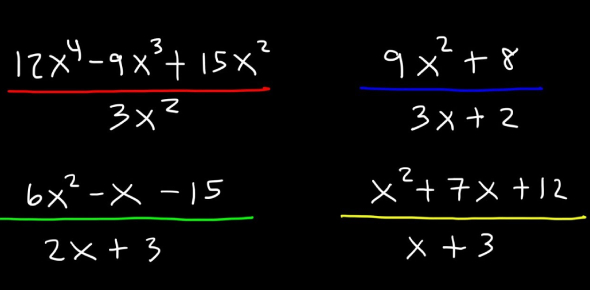# Algebra Quiz: Multiplication & Division Of Polynomials

10 Questions | Attempts: 354SettingsCheck out our online algebra quiz with questions on the multiplication and division of polynomials to test your knowlegde. With instant feedback, learn if you have got the right answer or not, in a single click.

• 1.
What is the product of -3x and -6x?
• A.

-18x2

• B.

18x

• C.

18x2

• D.

-18x

• 2.
What is the result in multiplying (-2x) to (4x2 + 3x - 3)?
• A.

8x3 – 6x2 + 6x

• B.

-8x3 – 6x2 + 6x

• C.

-8x3 + 6x2 - 6x

• D.

8x3 + 6x2 – 6x

• 3.
Find the product of (2n – 4) and (3n2 - 6n + 4).
• A.

6n3 – 24n2 + 32n – 16

• B.

6n3 + 24n2 – 32n – 16

• C.

6n3 – 24n2 – 32n + 16

• D.

6n3 + 24n2 + 32n + 16

• 4.
The length of the rectangle is 5m + 4 and the width is m - 9. What is the area of the rectangle?
• A.

5m2 – 41m – 36

• B.

5m2 + 41m – 36

• C.

5m2 – 41m + 36

• D.

5m2 + 41m + 36

• 5.
What is the area of a square if its side measures 9x - 4?
• A.

81x2 + 72x + 16

• B.

81x2 – 72x – 16

• C.

81x2 + 72x – 16

• D.

81x2 – 72x + 16

• 6.
If Anna would evaluate this expression, a7/a2  using the quotient rule, the what is the result?.
• A.

A-9

• B.

A-5

• C.

A5

• D.

A9

• 7.
What is the quotient of 27z5 divided by 26z3?
• A.

213z8

• B.

27/z2

• C.

Z/28

• D.

2z2

• 8.
If you divide 10x6 + 5x4 + 15x3 by 5x3, the result is                                            .
• A.

2x3 - x + 3

• B.

2x3 + x + 3

• C.

2x3 - x – 3

• D.

2x3 + x – 3

• 9.
Find the quotient of x3 + 5x2 + 2x – 8 divided by x + 2.
• A.

X2 + 3x – 4

• B.

X2 – 3x – 4

• C.

X2 + 3x + 4

• D.

X2 – 3x – 4

• 10.
What is the result if x3 – 5x2 + x + 15 will be divided by x – 3?
• A.

X2 – 2x + 5

• B.

X2 + 2x + 5

• C.

X2 – 2x – 5

• D.

X2 + 2x – 5

## Related TopicsBack to top
×

Wait!
Here's an interesting quiz for you.